Function Repository Resource:

# ColorGraphVertices

Color the vertices in a graph with no adjacent vertices sharing a color

Contributed by: Peter Cullen Burbery
 ResourceFunction["ColorGraphVertices"][graph] colors the vertices of graph with no adjacent vertices having the same color. ResourceFunction["ColorGraphVertices"][graph, colors] uses colors from the color list colors.

## Details

Every planar graph can have colors assigned to its vertices such that no adjacent vertices have the same color with at most four colors according to the four color theorem.
The vertices are colored using ColorData by default.
Deciding whether a graph can be colored with 3 or more colors is NP complete.

## Examples

### Basic Examples (1)

Color the vertices of the Petersen graph:

 In:=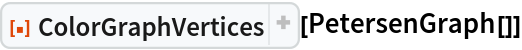Out=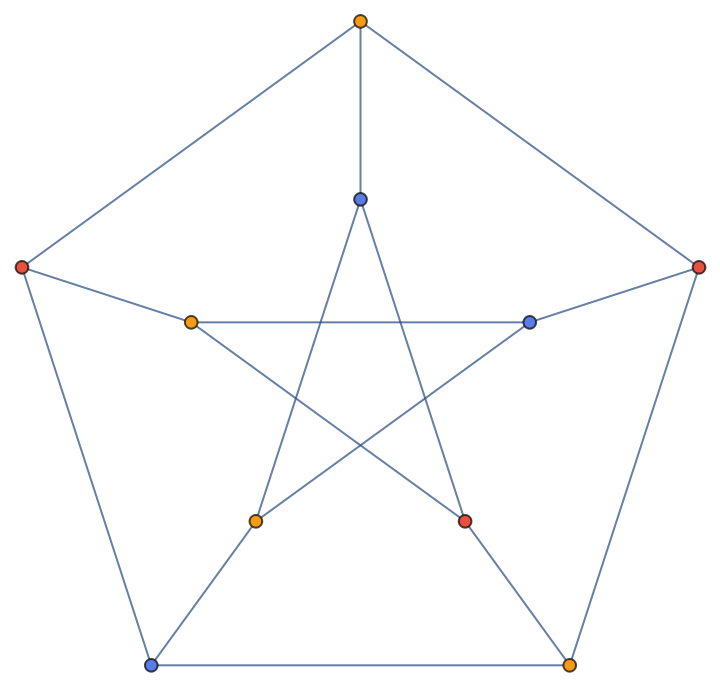### Scope (2)

Color the vertices of the Petersen graph with color list 105:

 In:=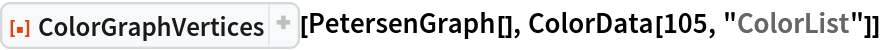Out=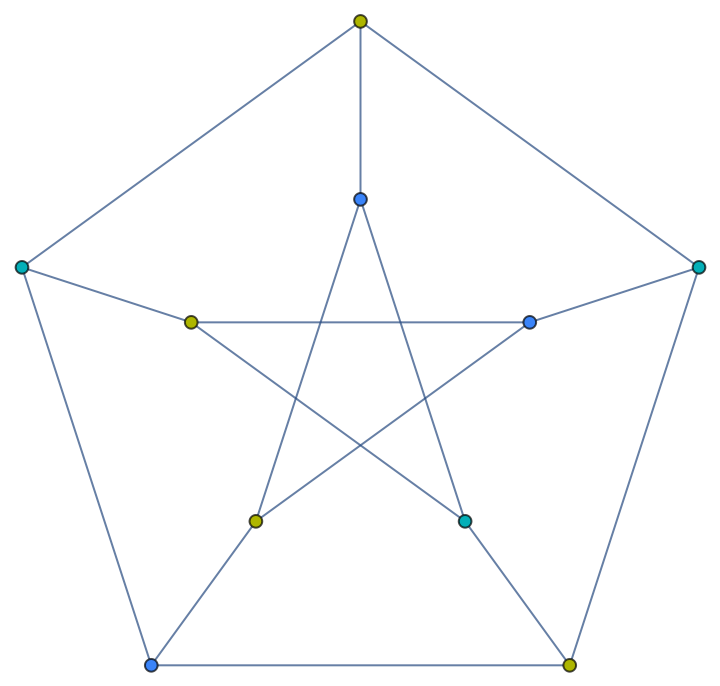Color the vertices of the Petersen graph with a random sample of crayola colors:

 In:=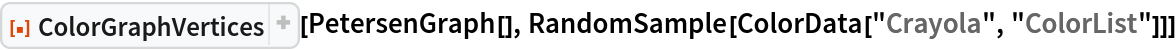Out=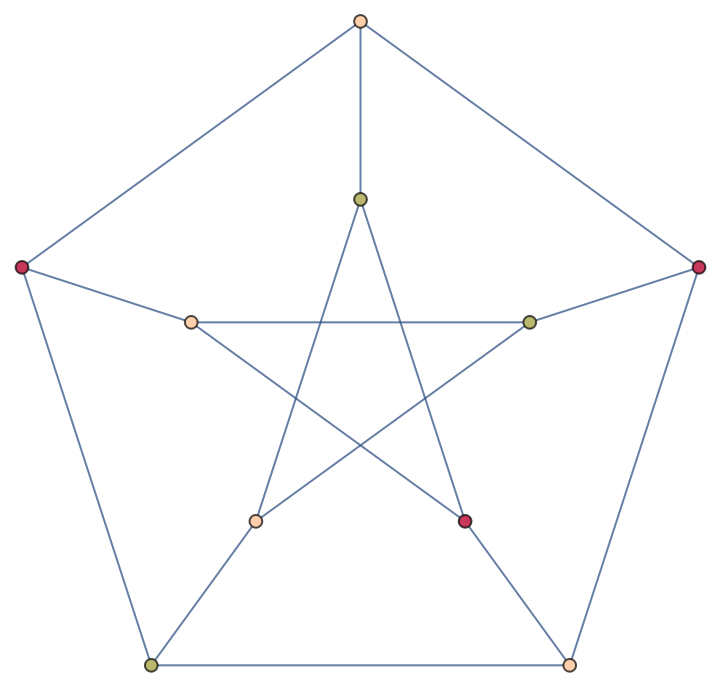Color the vertices of the Pappus graph:

 In:=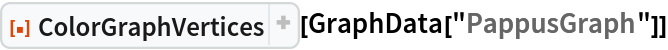Out=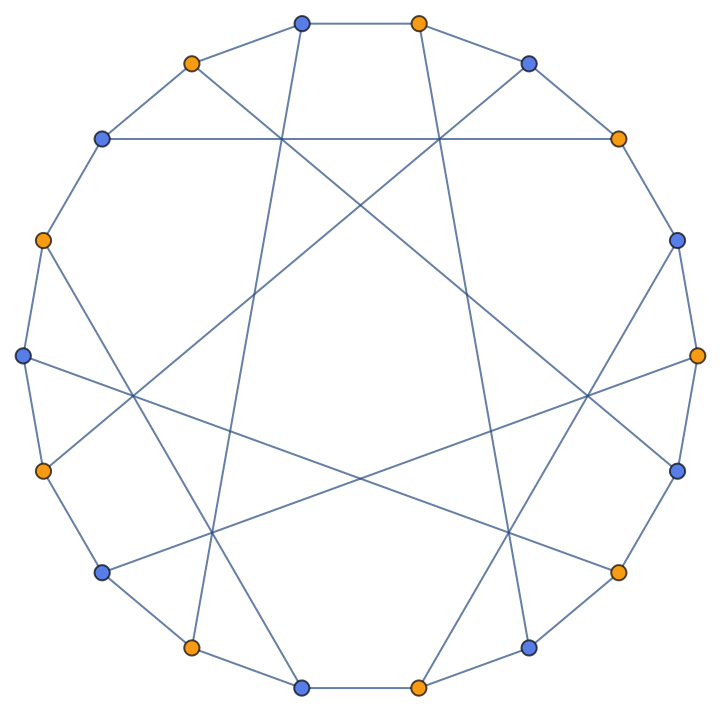### Options (1)

ColorGraphVertices takes the same options as Graph:

 In:=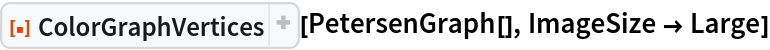Out=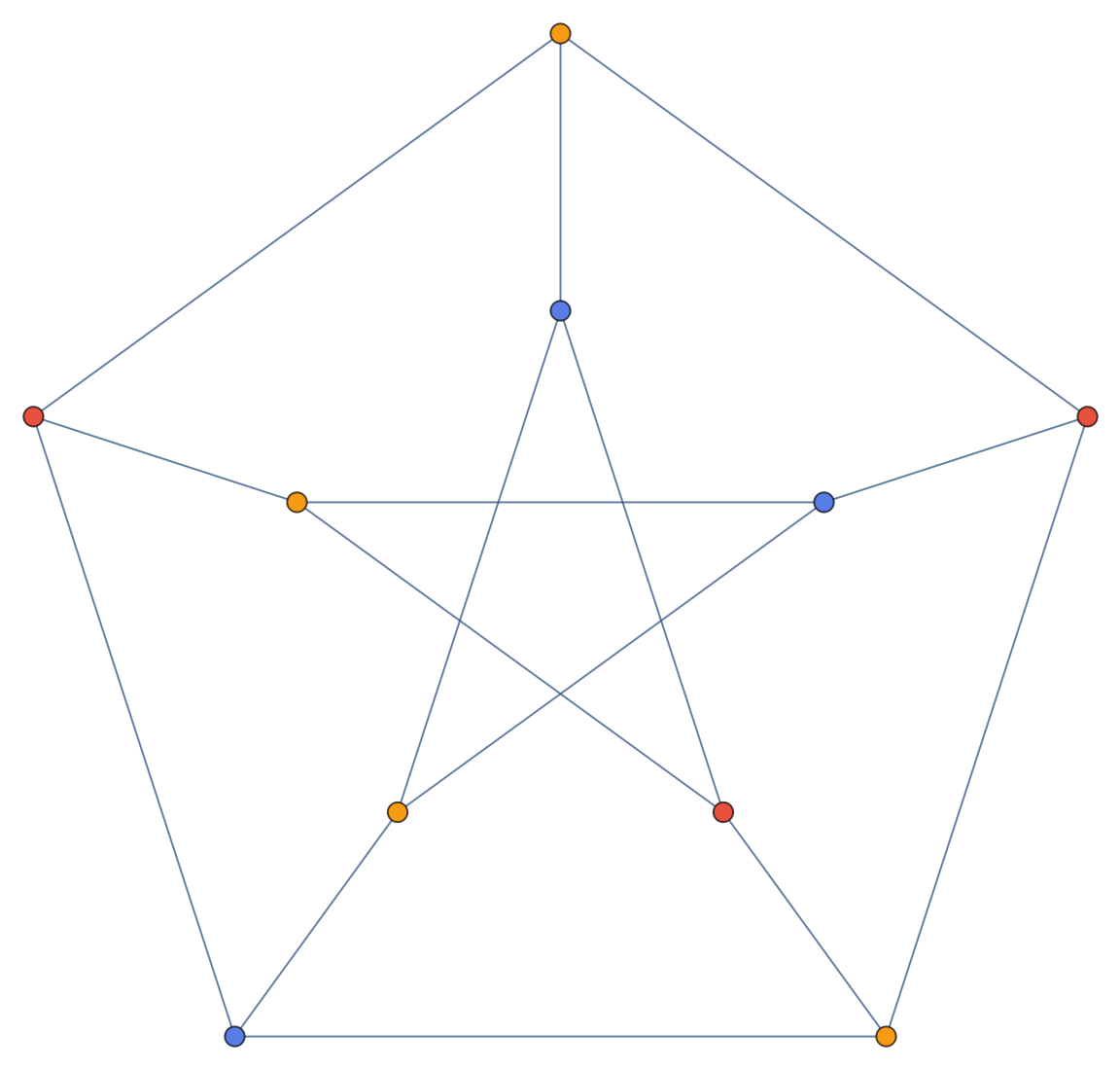### Properties & Relations (2)

Use ColorGraphVertices to color the vertices, or nodes of a graph or network, respectively:

 In:=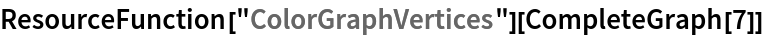Out=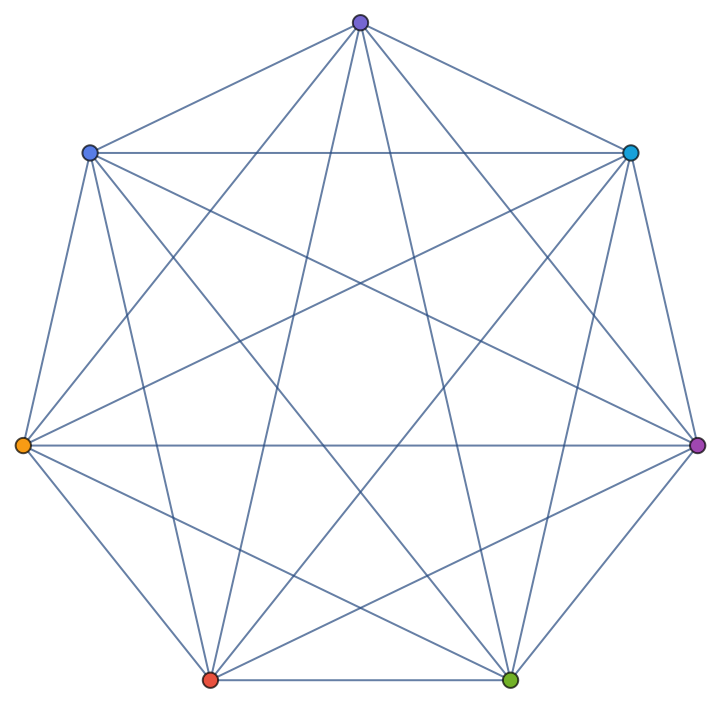One can also color the edges:

 In:=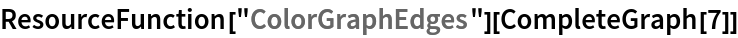Out=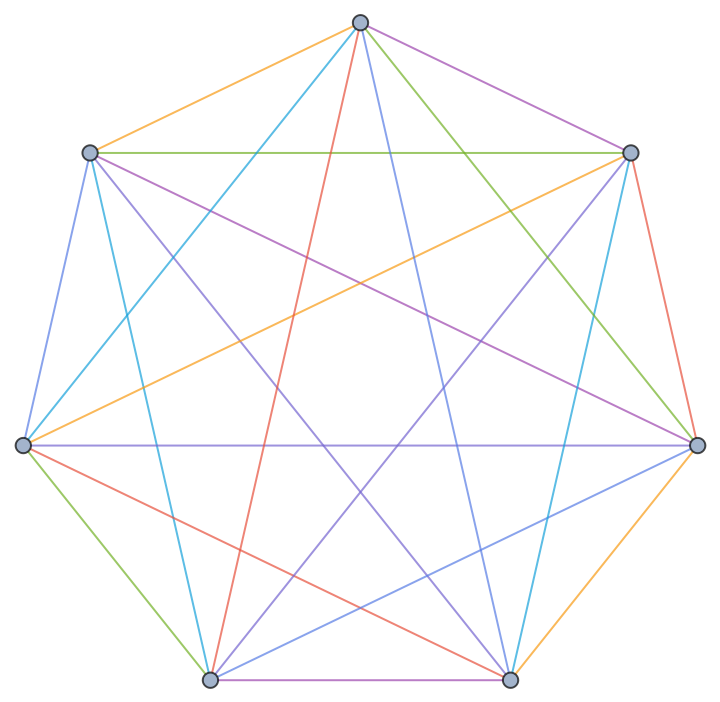The number of colors needed for the vertices is given by VertexChromaticNumber:

 In:=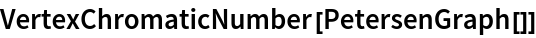Out=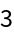Verify the cases with FindVertexColoring:

 In:=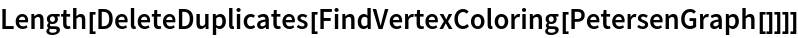Out=### Applications (3)

Color the vertices of the skeleton of the Császár polyhedron:

 In:=Out=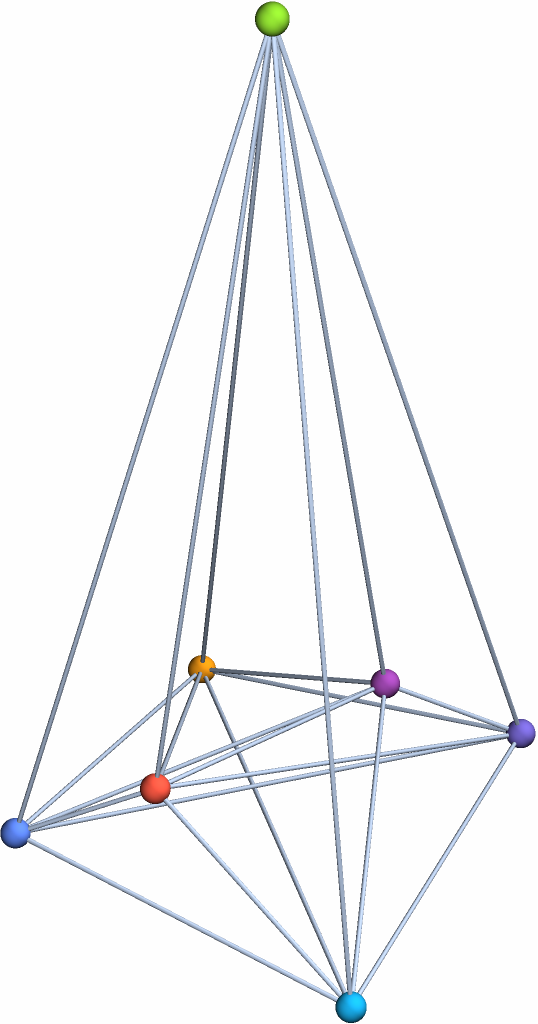Make a large random graph and color the vertices:

 In:=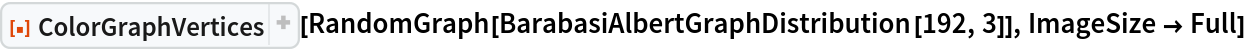Out=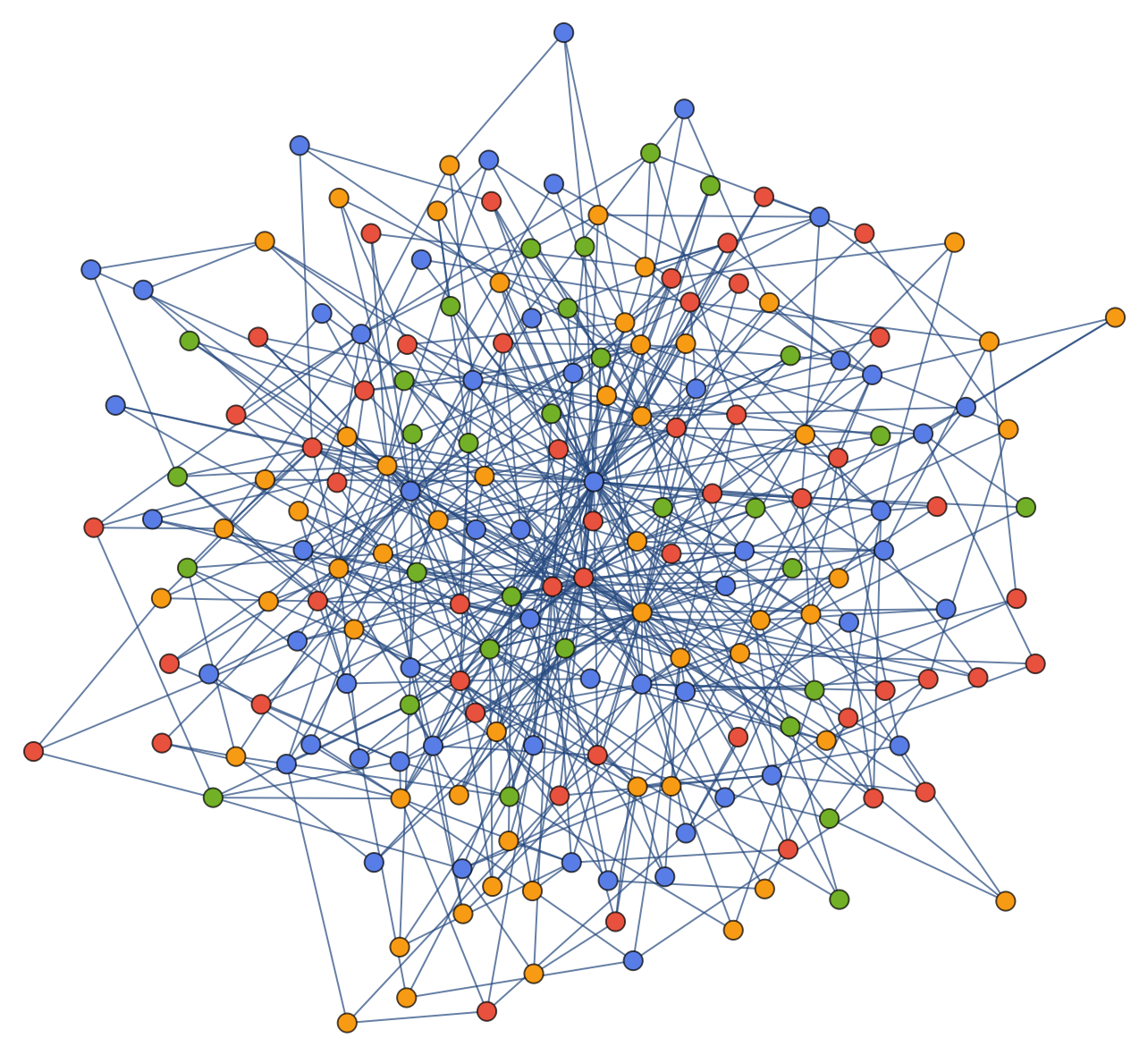Make a 3D buckyball graph with colors:

 In:=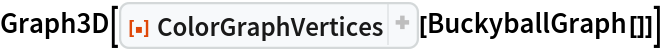Out=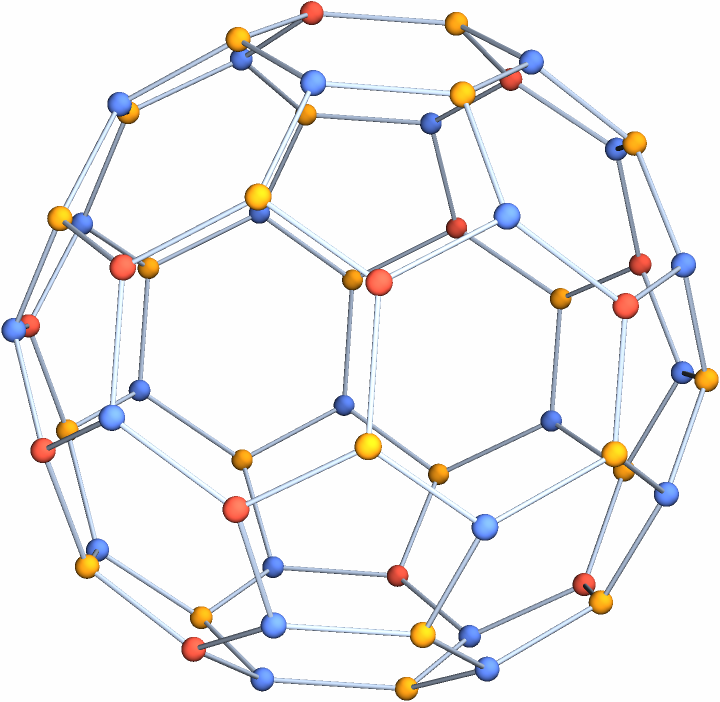Peter Burbery

## Requirements

Wolfram Language 12.3 (May 2021) or above

## Version History

• 1.1.0 – 13 July 2023
• 1.0.0 – 23 August 2022